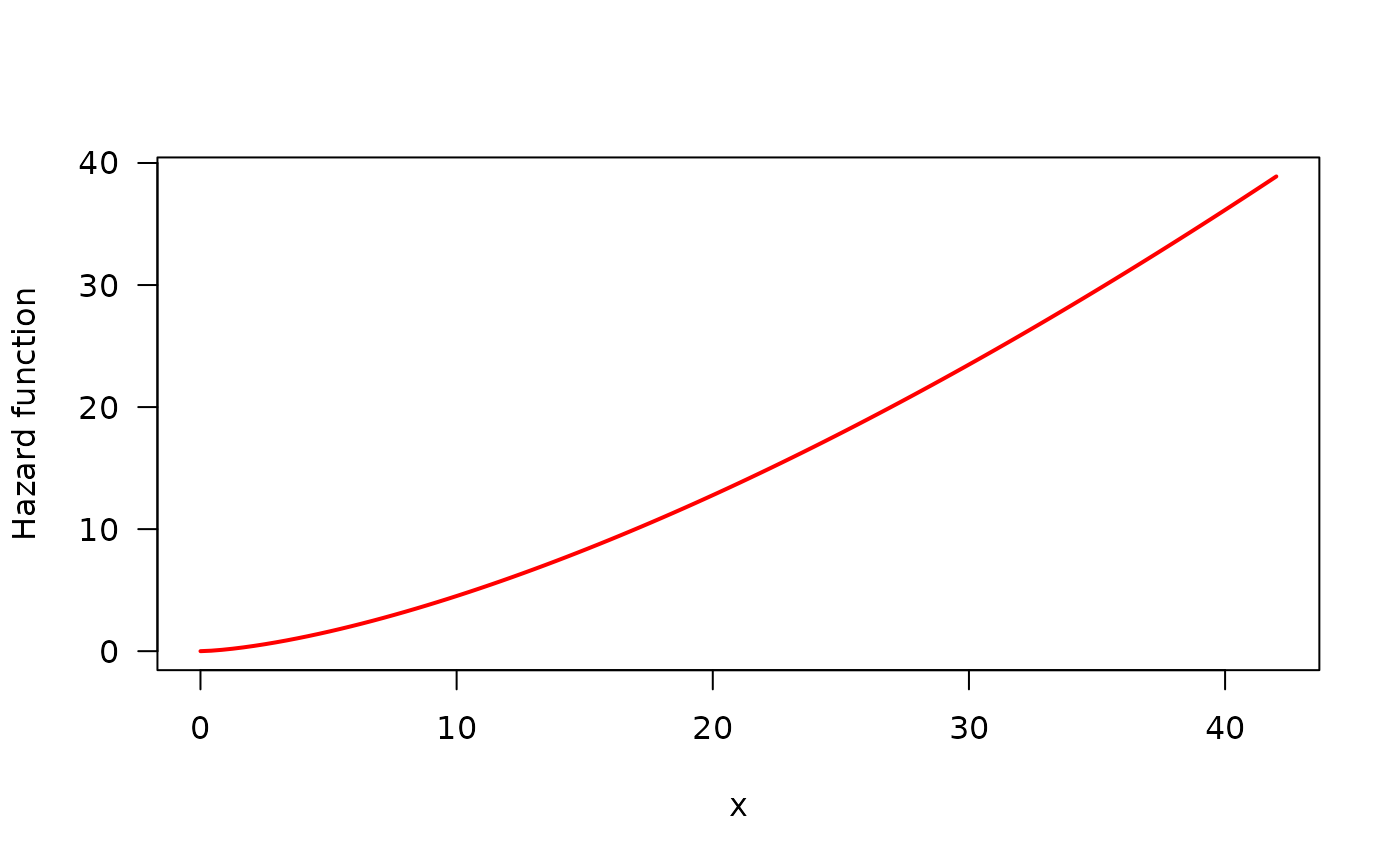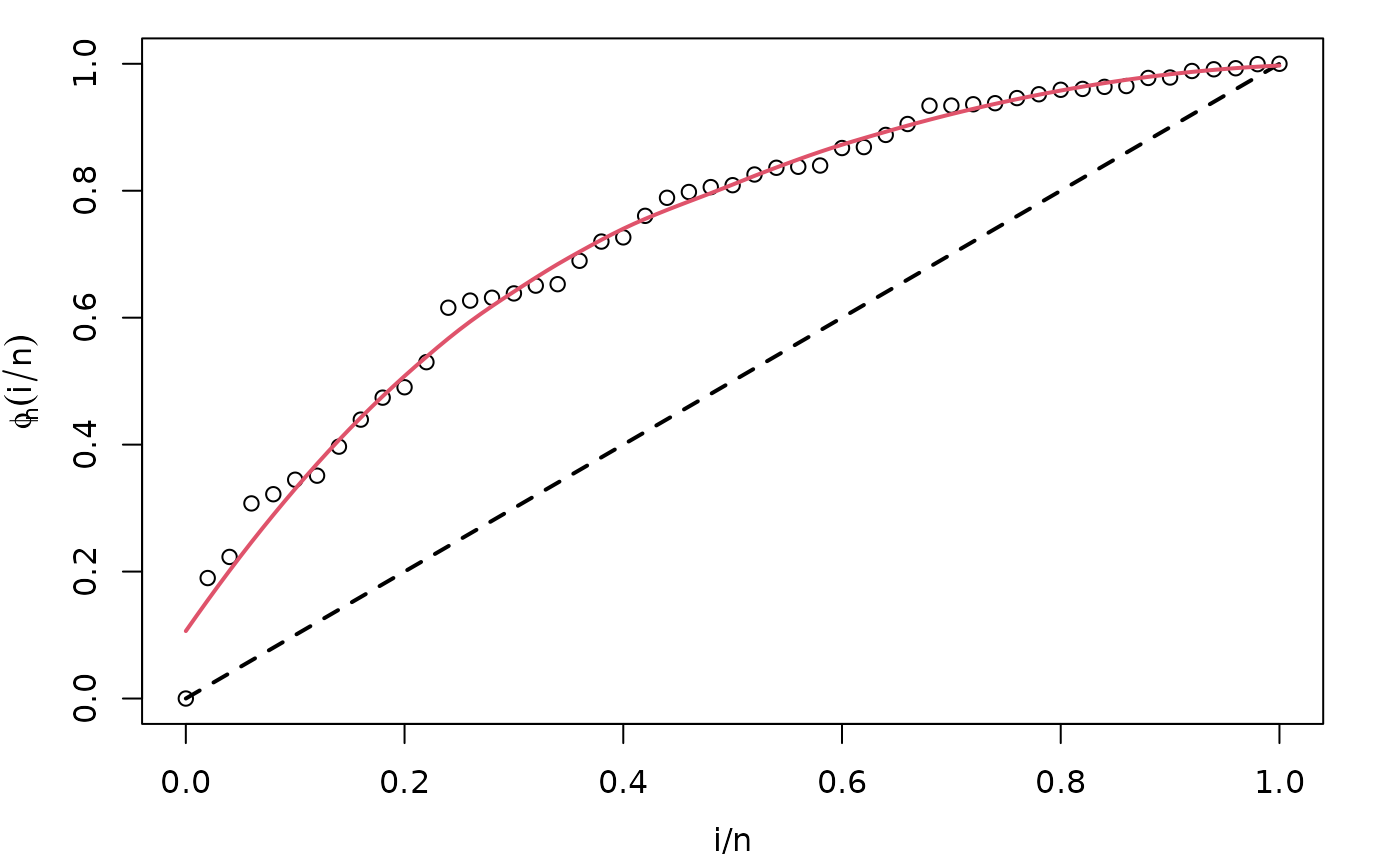Draws the empirical total time on test (TTT) plot and its non-parametric (LOESS) estimated curve useful for identifying hazard shape.

## Usage

# S3 method for HazardShape
plot(
x,
xlab = "i/n",
ylab = expression(phi[n](i/n)),
xlim = c(0, 1),
ylim = c(0, 1),
col = 1,
lty = NULL,
lwd = NA,
main = "",
curve_options = list(col = 2, lwd = 2, lty = 1),
par_plot = lifecycle::deprecated(),
legend_options = lifecycle::deprecated(),
...
)

## Arguments

x

an object of class initValOW, generated with TTT_hazard_shape.

xlab, ylab

titles for x and y axes, as in plot.

xlim

the x limits (x1, x2) of the plot.

ylim

the y limits (x1, x2) of the plot.

col

the colors for lines and points. Multiple colors can be specified. This is the usual color argument of plot.default.

lty

a vector of line types, see par for further information.

lwd

a vector of line widths, see par for further information.

main

a main title for the plot.

curve_options

a list with further arguments useful for customization of non-parametric estimate plot.

par_plot

(deprecated) some graphical parameters which can be passed to the plot. See Details section for further information.

legend_options

(deprecated) a list with fur further arguments useful for customization. See Details section for further information. of the legend of the plot.

...

further arguments passed to empirical TTT plot.

## Details

This plot complements the use of TTT_hazard_shape. It is always advisable to use this function in order to check the result of non-parametric estimate of TTT plot. See the first example in Examples section for an illustration.

## Author

Jaime Mosquera Gutiérrez jmosquerag@unal.edu.co

## Examples

library(EstimationTools)

#----------------------------------------------------------------------------
# Example 1: Increasing hazard and its corresponding TTT plot with simulated
# data
hweibull <- function(x, shape, scale) {
dweibull(x, shape, scale) / pweibull(x, shape, scale, lower.tail = FALSE)
}
curve(hweibull(x, shape = 2.5, scale = pi),
from = 0, to = 42,
col = "red", ylab = "Hazard function", las = 1, lwd = 2
)y <- rweibull(n = 50, shape = 2.5, scale = pi)
my_initial_guess <- TTT_hazard_shape(formula = y ~ 1)

par(mar = c(3.7, 3.7, 1, 2), mgp = c(2.5, 1, 0))
plot(my_initial_guess)#----------------------------------------------------------------------------